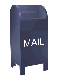## Basic Statistics

Over the last few years I have been collecting statistics books. I never took statistics in college since everyone I knew who took introductory statistics hated the class. Perhaps the reason for this is that statistics is poorly taught. Or perhaps people find statistics boring because they do not see how to apply statistics to applications that they find interesting.

Perhaps only sex or our survival focuses our fascination as much as money. Statistical mathematics are an indispensable tool in finance (e.g., portfolio analysis, risk analysis, statistical arbitrage). My interest in statistics arose from working on wavelet techniques for filtering and analyzing financial data (financial time series, like stock market daily close prices). Statistics provides a tool to understand whether a particular modeling technique may make money.

The rest of this web page consists of some notes on basic statistical functions. These statistical functions are not only useful for data analysis, but they are also building blocks for other techniques (like the calculation of the Hurst exponent). I've done little more than list the equations. In some cases I list the reference where I got the equation. See the reference list below for a list of statistics books. The C++ code that implements these functions is published here as well.

### Simple Linear Regression

Linear regression plots a line through a cloud of points. This is shown in the plot below.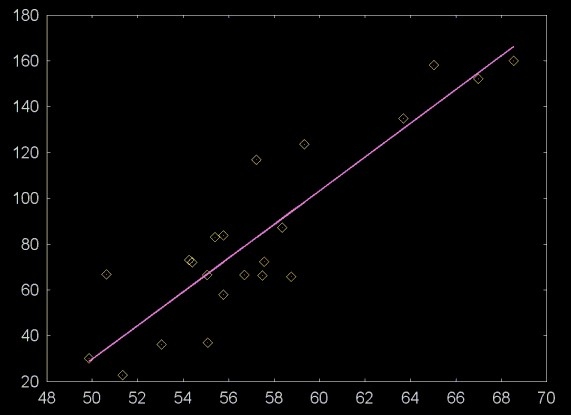The data points are from section 6.1 of Statistics Manual by Crow, Davis and Maxfield, Dover Press.

Given a set of values, X0...XN-1 and Y0...YN-1, linear regression calculates the constant coefficients a and b for the line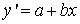The coefficient b (the slope of the line) is calculated with the equation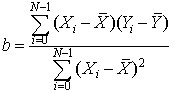Where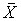is the mean of the x values and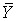is the mean of the y values.

The once the coefficient b is known, the coefficient a, the y-intercept, can be calculated using the equation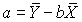When calculating the linear regression it is also important to calculate the error statistics, to give a measure of how closely the linear regression line fits the data. The standard deviation of the points around the regression line is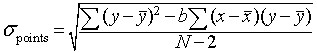From Cartoon Guide to Statistics and Introductory Statistics for Business and Economics

The standard error of the regression coefficient b is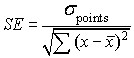From Cartoon Guide to Statistics and Introductory Statistics for Business and Economics

### Correlation

Calculation of the regression line is closely related to the calculation of the correlation. Two values are correlated when a change in one causes a change, in the same direction, in the other. For example, if a respected stock market analyst publishes a report that states that she believes that a stock will go up, the is some probability that the report will be positively correlated with a rise in the stock. Two values may be anti-correlated when a change in one causes a change in the opposite direction in the other. For example, a positive change in the polls for a politician, W, who favors deficits may be negatively correlated with the direction of US dollar currency futures, D. Or, put more simply as deficits go up, the value of the dollar in foreign exchange tends to go down. The correlation coefficient, r, has a value -1 <= r <= 1. A correlation of 0 means that the two values are unrelated. If 0 < r <= 1, then there is a positive correlation. If -1 <= r < 0, then there is a negative correlation.

The value R2 is calculated using the equation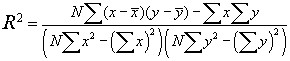From Statistics Manual by Crow et al

The correlation is the square root of R2, times the sign of the regression coefficient b (e.g., the slope of the line).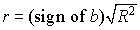### The Autocorrelation Function (ACF)

Autocorrelation is the correlation of a data set with itself, offset by n-values. For example, autocorrelation with an offset of 5 would correlate the data set {s0, s1, s2, s3 ... sn-5} is correlated with {s4, s5, s6, s7 ... sn}. The autocorrelation function is the set of autocorrelations with offsets 1, 2, 3, 4 .. limit, where limit <= n/2.

The equation for the autocorrelation function (ACF) is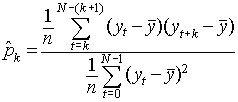This function is related to the autocovariance, with a forward step of k elements.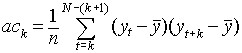The ACF equation I've used here (and implemented in software) is a modified version of the ACF given in Non-linear time series models in empirical finance by Philip Hans Frances and Kick van Kijk, Cambridge University Press, 2000, Section 2.2. Any errors are mine. I have gotten the same plots as Frances has published, using his data, so I have some reason to belive that my version is a correct translation.

### Standard Deviation

The standard deviation of a data distribution is calculated from the equation below. Here the standard deviation is calculated from the square root of the "unbiased" estimate of the variance (where n-1 rather than n is used as a divisor). Some statistics text books use a form of the standard deviation where n is the devisor, but calculators and statistics software use the version shown here. Again, in the equation below,is the mean.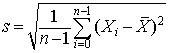### Math Packages vs. Writing Your Own Code

So far I've implemented all the statistics functions I've used in either C++ or Java. This has the advantage that I've developed a fairly complete understanding of the functions I've implemented. Math packages like MatLab, Mathematica and S+ provide support for basic statistics and include packages that support additional functions like wavelets. These packages also provide some level of support for plotting the results. So far I have not been able to justify the significant license fees for this software. Although implementing software forces a better understanding of the statistical functions being used, it is very time consuming. These statistical functions are a tool for what I'm trying to acomplish, rather than an end in themselves. So better math support is becoming increasingly attractive. There are three open source (or "public domain") packages that look interesting:

1. To quote the Octave web page:

GNU Octave is a high-level language, primarily intended for numerical computations. It provides a convenient command line interface for solving linear and nonlinear problems numerically, and for performing other numerical experiments using a language that is mostly compatible with Matlab. It may also be used as a batch-oriented language.

MatLab seems to be one of the most, if not the most, popular mathematics environments. In the book Ripples in Mathematics, which I used for reference for much of my wavelet work, many of the examples are given in MatLab code.

2. R is a free software version of the S+ mathematics environment. I used S+ for work I did on wavelets while I was working at Prediction Company. The version of S+ we were using was heavily modified for the local environment (Prediction Company has a source license). This made it difficult to migrate to new versions of S+. So I'm not sure whether my experience with S+ is representative of the current product. I was not very happy with the S+ graphics environment. Also, S+ seems to be used much less than MatLab.

Although S+ may have limitations, the R Project does look interesting. The documentation looks good and it has a more "professional feel" than Octave.

3. Dataplot has been developed by the US National Institute of Standards and Technology. The software started being developed by James J. Filliben in 1978. It now includes a fairly sophisticated plotting and data analysis environment. The software is supported on virtually every major platform.

### Copyright and use of the associated software

You may use this source code without limitation and without fee as long as you include:

This software was written and is copyrighted by Ian Kaplan, Bear Products International, www.bearcave.com, 2001.

This software is provided "as is", without any warranty or claim as to its usefulness. Anyone who uses this source code uses it at their own risk. Nor is any support provided by Ian Kaplan and Bear Products International. Please send any bug fixes or suggested source changes to:
```     iank@bearcave.com
```

### Software

The C++ source code that implements the basic statistics functions described here (along with C++ code to calculate the probability density function, which is not described here) can be downloaded by clicking here. This file is in uncompressed tar format.

The documentation for this source code, generated by Doxygen can be found here.

### Books

• The Cartoon Guide to Statistics by Larry Gonick and Woollcott Smith, Harper Collins, 1993

I'm always a bit embarrassed to list this book as a reference, since the title sounds so non-serious. But this is one of the best concise introductions to statistics that I've found. The book is an easy introduction to basic statistics, although it can be a bit shallow. The Cartoon Guide to Statistics includes many of the basic statistics equations.

• Introductory Statistics for Business and Economics Fourth Edition, Thomas H. Wonnacott and Ronald J. Wonnacott, John Wiley and Sons 1990

This is an excellent statistics book which provides a clear introduction with out shying away from equations, but avoiding proofs. This book has more depth than The Cartoon Guide to Statistics and covers topics like multiple linear regression.

• "Social Science" majors usually have to take a statistics course as part of their degree requirements. In some cases they choose their major because they did not like (or do well in) college math. So there are a statistics books for social science majors that approach statistics without much in the way of equations. While these books usually provide a very readable introduction to statistics, they make poor references in many cases, because in a reference you want to find the equation. Two "social science" statistics books that I've purchased are:

1. Statistics: A Bayesian Perspective by Donald A. Berry, Wadsworth, 1996.

This book is readable and provides basic statistics equations, in some cases. But like the book below it avoids any notation that might be encountered in calculus (e.g.,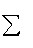).

2. Statistics, Third Edition, by Freedman, Pisani and Purves, Norton, 1998

This book is the worst of the two. By staying away from equations, the discussion is needlessly obscure. You get derivations like this one: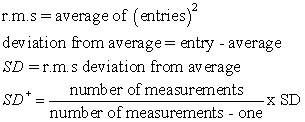This attempts to describe the unbiased standard deviation. Clearly the equation above is simpler. I ended up getting rid of this book.

• Principles of Statics M.G. Bulmer, Dover Press, 1979

Frequently I want a quick discussion of a statistical technique, where the author is not afraid to give the equations and even use basic calculus. However, I don't want the "statistics for math majors" which is heavy on proofs. This book nicely fits my requirements. In some cases the explainations are a bit obscure and I've had to read some sections a few times to understand the material. Dover Press has been reprinting math books at very reasonable prices, so the book is reasonably priced as well.

• Statistics Manual by Crow, Davis and Maxfield, Dover Press,1960

This is an even more concise catalog of statistical techniques. Each technique includes a brief explanation and the basic equations. This book was written before computing power was either affordable or widely available. Some of the simplifications for the equations make sense if you are doing the calculation by hand, but offer no real advantage in software. As with all Dover math books, this is reasonably priced.

• Against the Gods: The Remarkable Story of Risk by Peter L. Bernstein, 1996

This excellent books discusses the origins of statistics (the gambling salons in Europe) and practical applications of statistical theory to insurance and finance.

• Capital Ideas: The Improbable Origins of Modern Wall Street by Peter L. Bernstein, 1993

Another great book by Peter Bernstein. This book proves one of the best overviews of modern economic market theory and quantitative finance (which includes statistical techniques). Quantitative finance is driven by a desire for profit, so it is a field that moves rapidly as very smart people apply leading edge mathematical techniques to market trading and modeling. As a result, Capital Ideas is somewhat out of date, but it still is worth reading.

### Web Based References

• Fast Median Finding Algorithms

When George W. Bush was selling the second of his tax cuts to the people of the United States and their representatives in Congress he talked about the average tax cut that a US family would receive. The problem with the number quoted by Bush was that while a high income family would receive a large tax cut, a low income family would receive very little. What "W" should have used was the median, rather than the mean, or average. This would have yielded a number much closer to what most families would actually receive.

If we have an ordered list of numbers, the median is the number in the middle. If the number of values is even, then the median is the value between the two numbers closest to the middle. The obvious way to find the median is to sort the numbers. A fast sort algorithm has a time complexity of On log(n). Once the numbers are sorted we can directly find the median at the middle index of the number list.

CAR Hoare, who invented the Quick Sort algorithm, and developed a lot of the theory of communicating sequential processes also developed a fast median finding algorithm that will find the median in O(n) time. In An Efficient Algorithm for the Approximate Median Selection Problem Battiato et al, October 1999 (in pdf format) has an algorithm that is even faster (basicly N).

• Chi Square Tutorial (PDF) by Prof. Jeff Connor-Linton, Georgetown University

### Acknowledgments

I want to thank Keith Dyke who, in a time which seems increasingly long ago, in a land far away from where I am now, patiently explained basic statistics to me and helped me gain some facility with the S+ statistics package.

Ian Kaplan
May 2003
Revised: